# Find this unknown angle in the triangle

• MHB
tato1982
Find the unknown angle in the triangle. It is quite an interesting task. I will put a trigonometric solution, Mabra I wonder how it opens geometrically. Help someone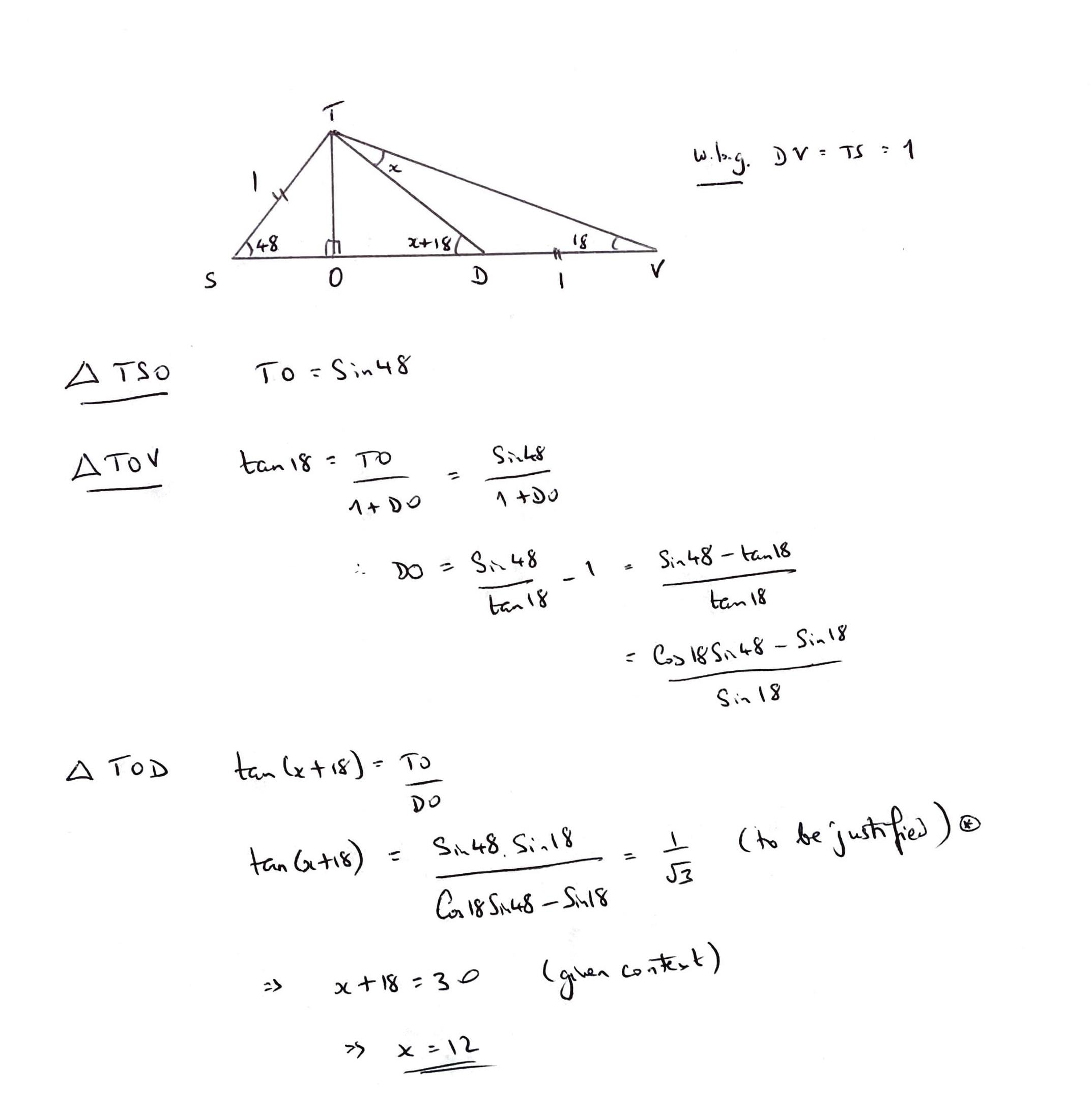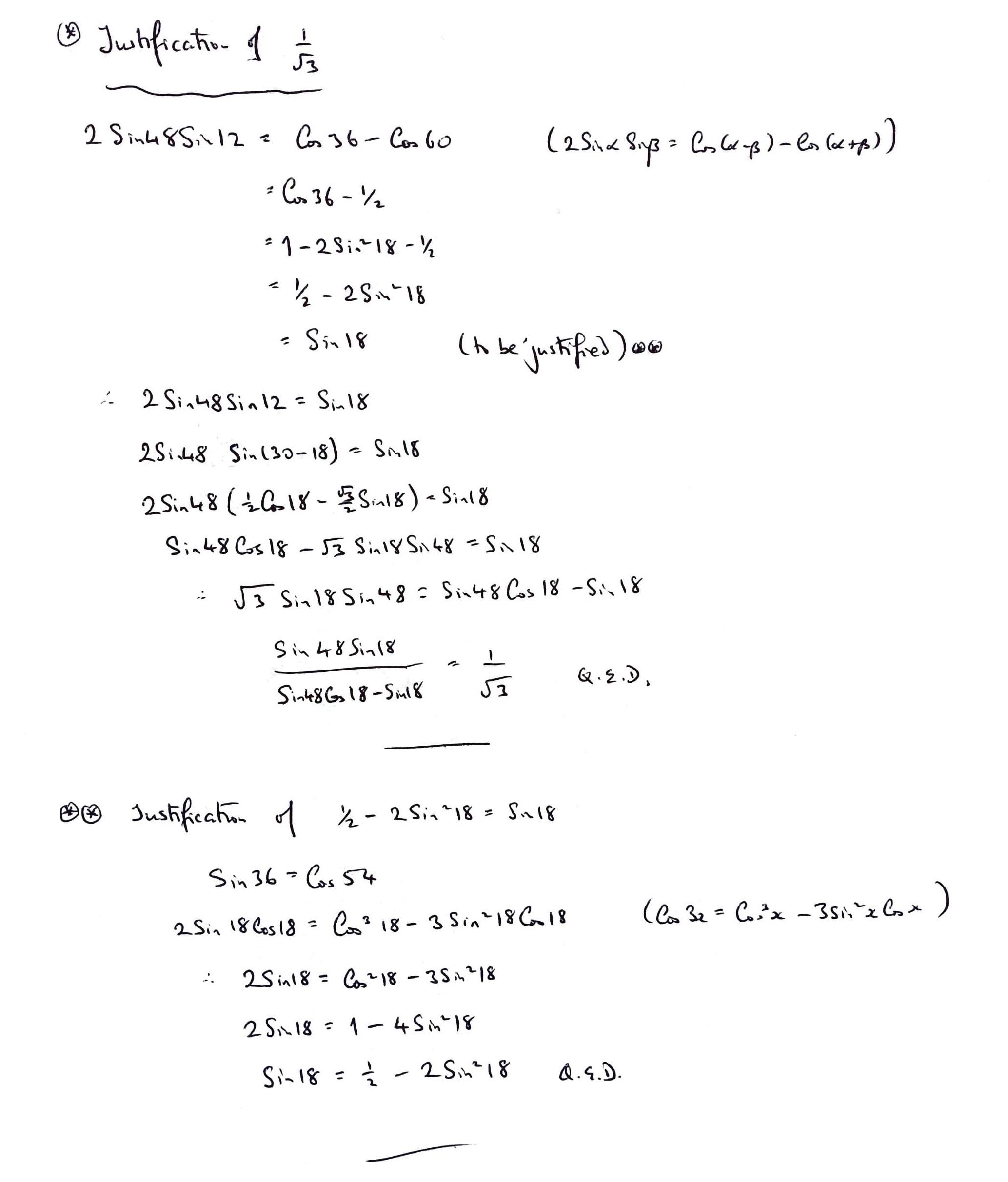Last edited by a moderator:
•Gold Member
Find the unknown angle in the triangle. It is quite an interesting task. I will put a trigonometric solution, Mabra I wonder how it opens geometrically. Help someoneView attachment 312479View attachment 312480
I am getting the same solutions, i.e ##x=12## and ##y=60## i only used pythagoras theorem, SOHCAHTOA and sine rule in my working...no trigonometric identities like you have used.

Homework Helper
You can solve: $$\sin x \sin48=\sin18\sin(x+18)$$ Wolfram Alpha 'choked'on it for some reason but the manual solution is fairly straightforward.

•Wrichik Basu, chwala and Charles Link
Homework Helper
Or get to the same place by applying tan rule directly to triangle TDV: $$\tan x = \frac{VD \sin 18}{TV-VD \cos 18}$$ with ##VD=1## and ##TV=\frac{\sin 48}{\sin 18}##

•Homework Helper
••Homework Helper
For the record: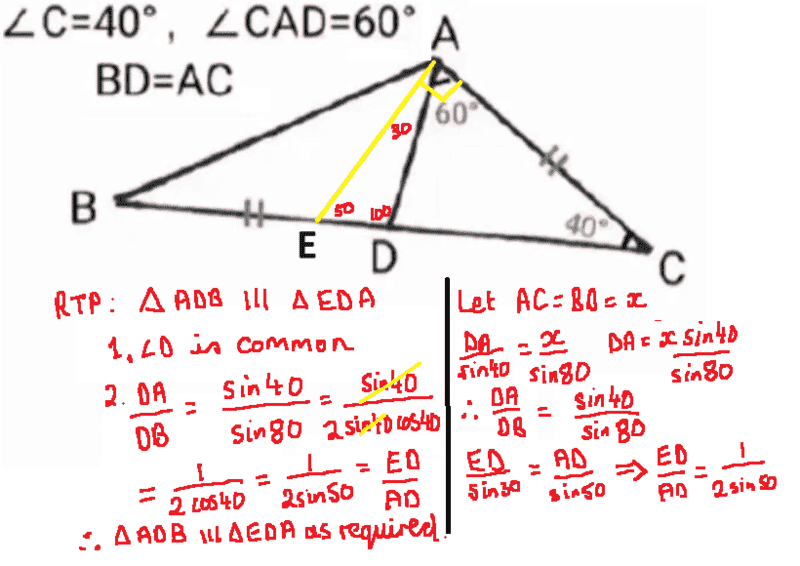•Gold Member
Find the unknown angle in the triangle. It is quite an interesting task. I will put a trigonometric solution, Mabra I wonder how it opens geometrically. Help someoneView attachment 312479View attachment 312480

Am trying to follow the working here; the trig identities are correct but a bit confusing ...particularly on the line...

##2\sin 18^0\cos18^0=\cos^{3}18^0 - 3\sin^{2}18^0 \cos 18^0## ...

##\sin 36^0= \cos 54^0##

therefore using;

##\cos 3θ=\cos (2θ+θ)= \cos^{3}θ- 3\sin^{2}θ \cos θ##

we shall have,

##\cos 54^0= \cos (36^0+18^0)=\cos^{3}18^0 - 3\sin^{2}18^0 \cos 18^0##

...

Last edited:
Gold Member
Find the unknown angle in the triangle. It is quite an interesting task. I will put a trigonometric solution, Mabra I wonder how it opens geometrically. Help someoneView attachment 312479View attachment 312480
Allow me to ask this ; Consider the highlighted part in red...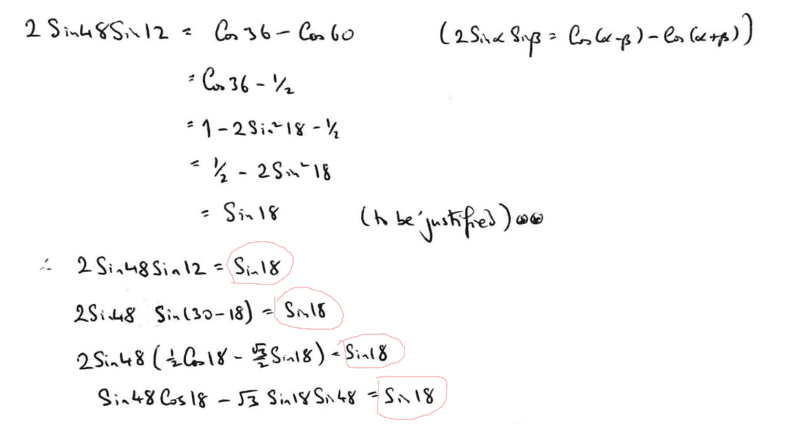...you plugged in ##\sin 18^0## on the rhs and used it to show your justification...i do not think that's the correct approach for justifying a trigonometric identity. In my thinking we need to use only the lhs of the identity to realise/prove the envisaged right hand side.

Staff Emeritus
Homework Helper
Gold Member
Allow me to ask this ; Consider the highlighted part in red...

View attachment 322065

...you plugged in ##\sin 18^0## on the rhs and used it to show your justification...i do not think that's the correct approach for justifying a trigonometric identity. In my thinking we need to use only the lhs of the identity to realise/prove the envisaged right hand side.
I doubt that you'll get an answer back.

This thread is @tato1982 ' s one and only post.

•chwala
Gold Member
You can solve: $$\sin x \sin48=\sin18\sin(x+18)$$ Wolfram Alpha 'choked'on it for some reason but the manual solution is fairly straightforward.
This was a nice one...almost choked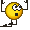...i think the intention was for the OP to continue with the hint; but having said that i ended up with;

##\tan x = \dfrac{\sin^2 a}{\sin b-\sin a\cos a}=0.2125566##

with ##a## and ##b## being integer values. We shall end up with,

##x= \tan^{-1} [0.2125566]= 12^0##.

•neilparker62
Homework Helper
Gold Member
I was able to follow what the OP did, and he later justified a couple of steps which he assumed to be correct, because he needed the result to complete another step. The OP asked if anyone has a simpler way of solving this, and I haven't been able to come up with one.

It is very easy to get a numerical result, but proving that it is exactly 12 degrees I found to be much more difficult.

e.g. You can get TV immediately from the law of sines, and then TD from the law of cosines, and from that you can get ##\cos{x} ## or ## \sin{x} ## with the law of cosines or the law of sines. I don't see a simple two or three step process though to get that ## x=12 ## degrees.

Last edited:
•neilparker62 and chwala
Homework Helper
I was able to follow what the OP did, and he later justified a couple of steps which he assumed to be correct, because he needed the result to complete another step. The OP asked if anyone has a simpler way of solving this, and I haven't been able to come up with one.

It is very easy to get a numerical result, but proving that it is exactly 12 degrees I found to be much more difficult.

e.g. You can get TV immediately from the law of sines, and then TD from the law of cosines, and from that you can get ##\cos{x} ## or ## \sin{x} ## with the law of cosines or the law of sines. I don't see a simple two or three step process though to get that ## x=12 ## degrees.
I used the tan rule to get to 12 degrees. But yes - it's a numerical answer which does not exactly prove 12 degrees. Have tried all sort of geometric 'tricks' to no avail. However I have been able to determine a surd expression for tan(12) (degrees) as sin(30-18)/cos(30-18) and then show it is equivalent to the expression I got using the tan rule. Well I will get to post that but it's quite a lot of Latex and not really a "simple 2 or 3 step" process!

•Homework Helper
##18^{\circ}## is a Pentagon related angle along with ##36^{\circ}, 54^{\circ}## and ##72^{\circ}##. All of these are characterised by their connection to the golden ratio ##\phi \approx 0.618 ##.

##\sin18^{\circ}=\frac{\phi}{2}##
##\sin36^{\circ}=\frac{\sqrt{2-\phi}}{2}##
##\sin54^{\circ}=\frac{1}{2\phi}##
##\sin72^{\circ}=\frac{\sqrt{3+\phi}}{2}##

For this application we'll need ##\sin18^{\circ}## and ##\sin72^{\circ}=\cos18^{\circ}##. And we'll need the golden ratio specific relationship: ##1-\phi^2=\phi## or alternatively ##1 - \phi = \phi^2##.

Last edited:
•chwala
Homework Helper
$$\tan 12^{\circ}=\tan (30-18)^{\circ}=\frac{\sin (30-18)^{\circ}}{\cos (30-18)^{\circ}}$$ $$=\frac{\sin 30^{\circ} \cos 18^ {\circ}-\cos 30^{\circ} \sin 18 ^{\circ} }{ \cos 30^{\circ} \cos 18^{\circ}+\sin 30^{\circ} \sin 18^{\circ}}$$ $$=\frac{\frac{1}{2} \frac{\sqrt{3+\phi}}{2} -\frac{\sqrt 3}{2} \frac{\phi}{2}} {\frac{\sqrt 3}{2} \frac{\sqrt{3+\phi}}{2} +\frac{1}{2} \frac{\phi}{2}}$$ $$=\frac{\sqrt{3+\phi} - \sqrt{3} \phi } {\sqrt{3} \sqrt{3+\phi} + \phi}$$
Now we "rationalise" the numerator by multiplying top and bottom by ##\sqrt{3+\phi} +\sqrt{3}\phi##:
$$=\frac{3 + \phi - 3\phi^2}{\sqrt{3}(3+\phi)+3\phi\sqrt{3+\phi}+\phi\sqrt{3+\phi}+\sqrt{3}-\sqrt{3}\phi}$$ $$=\frac{4\phi}{4\sqrt{3}+4\phi \sqrt{3+\phi}} = \frac{\phi}{\phi\sqrt{3+\phi}+\sqrt{3}}$$

•chwala
Homework Helper
From post #4 we had in effect: $$\tan(x) = \frac{\sin^2 18^{\circ}}{\sin 48^{\circ}-\sin 18^{\circ} \cos 18^{\circ}} = \frac{\sin^2 18^{\circ}}{\sin (30+18)^{\circ}-\sin 18^{\circ} \cos 18^{\circ}}$$ $$=\frac{\frac{\phi^2}{4}} {\frac{1}{2}\frac{\sqrt{3+\phi}}{2}+\frac{\sqrt{3}}{2} \frac{\phi}{2} - \frac{\phi}{2} \frac{\sqrt{3+\phi}}{2} } = \frac{\phi^2} {\phi^2 \sqrt{3+\phi} + \sqrt{3} \phi }$$ $$=\frac{\phi}{\phi \sqrt{3+\phi} + \sqrt{3} }$$ Since this is the same expression we obtained above for ##\tan 12^{\circ}##, we may reasonably conclude that ##x = 12^{\circ}##.

••Gold Member
From post #4 we had in effect: $$\tan(x) = \frac{\sin^2 18^{\circ}}{\sin 48^{\circ}-\sin 18^{\circ} \cos 18^{\circ}} = \frac{\sin^2 18^{\circ}}{\sin (30+18)^{\circ}-\sin 18^{\circ} \cos 18^{\circ}}$$ $$=\frac{\frac{\phi^2}{4}} {\frac{1}{2}\frac{\sqrt{3+\phi}}{2}+\frac{\sqrt{3}}{2} \frac{\phi}{2} - \frac{\phi}{2} \frac{\sqrt{3+\phi}}{2} } = \frac{\phi^2} {\phi^2 \sqrt{3+\phi} + \sqrt{3} \phi }$$ $$=\frac{\phi}{\phi \sqrt{3+\phi} + \sqrt{3} }$$ Since this is the same expression we obtained above for ##\tan 12^{\circ}##, we may reasonably conclude that ##x = 12^{\circ}##.
Boss! Impressive!

•neilparker62
Homework Helper
Gold Member
The way I have is a little simpler, but still slightly complex:
We need to show that ## 2 \sin(48) \sin(12)=\sin(18) ##, (that will make the one triangle a 30-60-90, and the angle x to be 12 degrees ), so that
## 2 \sin(30+18) \sin(30-18)=\sin(18) ##, and expanding with trig identity
##2 ((1/4)\cos^2(18)-(3/4) \sin^2(18))= \sin(18) ## so that
## 4 \sin^2(18)+2 \sin(18)-1=0 ##.
We get that ## \sin(18) ## must satisfy the post 14 expression, and the identity is shown to be correct.

The following I found in a google: (to get the expression in post 14)
Let 18 degrees=## a ##.
Then
## 2a=90-3a ##.
## \sin(2a)=\sin(90-3a)=\cos(3a) ##.
Next:
##2 \sin(a) \cos(a)=4 \cos^3(a)-3 \cos(a)=\cos(a)(4-4 \sin^2(a)-3) ##.
So that:
## \cos(a)(4 \sin^2(a)+2 \sin(a)-1)=0 ##.
Can then solve for ## \sin(a) ##.

Last edited:
•chwala
Homework Helper
We can also find expressions for TO ##( \sin 48^\circ)## and TD ##( \frac{\sin 48^\circ \cos 18^\circ}{\sin 18^\circ}-1)##. Then we should have ##\frac{TD}{TO}=\cot 18^{\circ} - \csc 48^{\circ} = \sqrt{3}## and I will leave proof of this identity to the reader.

Have fun!

•chwala
Homework Helper
Find the unknown angle in the triangle. It is quite an interesting task. I will put a trigonometric solution, Mabra I wonder how it opens geometrically. Help someoneView attachment 312479View attachment 312480
Looks good - certainly a very good exercise for your trig skills! I've tried all sorts of "tricks" with geometry but as yet cannot find a geometric solution.

Gold Member
##18^{\circ}## is a Pentagon related angle along with ##36^{\circ}, 54^{\circ}## and ##72^{\circ}##. All of these are characterised by their connection to the golden ratio ##\phi \approx 0.618 ##.

##\sin18^{\circ}=\frac{\phi}{2}##
##\sin36^{\circ}=\frac{\sqrt{2-\phi}}{2}##
##\sin54^{\circ}=\frac{1}{2\phi}##
##\sin72^{\circ}=\frac{\sqrt{3+\phi}}{2}##

For this application we'll need ##\sin18^{\circ}## and ##\sin72^{\circ}=\cos18^{\circ}##. And we'll need the golden ratio specific relationship: ##1-\phi^2=\phi## or alternatively ##1 - \phi = \phi^2##.
looks brilliant man! but just a question- why did you have to come up with the golden ratio specific relationship 'transforms' i.e
##1-\phi^2=\phi## or alternatively ##1 - \phi = \phi^2##.

For convenience/aesthetics i suppose...of course i can also see that you used the property ##\cos^2 ∅ + \sin^2∅=1## to find ##\cos 18^0=\dfrac{\sqrt {4-∅^2}}{2}=\dfrac{\sqrt {4-(1-∅)}}{2}=\dfrac{\sqrt {3+∅)}}{2}## according to your transform.

Last edited:
Homework Helper
The following I found in a google: (to get the expression in post 14)
Let 18 degrees=## a ##.
Then
## 2a=90-3a ##.
## \sin(2a)=\sin(90-3a)=\cos(3a) ##.
Next:
##2 \sin(a) \cos(a)=4 \cos^3(a)-3 \cos(a)=\cos(a)(4-4 \sin^2(a)-3) ##.
So that:
## \cos(a)(4 \sin^2(a)+2 \sin(a)-1)=0 ##.
Can then solve for ## \sin(a) ##.
Apart from "Uncle Google" , you may also refer to Euclid XIII 9 !

•Homework Helper
looks brilliant man! but just a question- why did you have to come up with the golden ratio specific relationship 'transforms' i.e

For convenience/aesthetics i suppose...of course i can also see that you used the property ##\cos^2 ∅ + \sin^2∅=1## to find ##\cos 18^0=\dfrac{\sqrt {4-∅^2}}{2}=\dfrac{\sqrt {4-(1-∅)}}{2}=\dfrac{\sqrt {3+∅)}}{2}## according to your transform.
Yes - that's one instance it was needed. I also used it in the derivation of the expression I gave for ##\tan 12^\circ##. And in showing this was equivalent to the other expression I obtained in post #4. You'll also need it if you pick up the challenge above to show ##\frac{TD}{TO}=\sqrt 3##.

It's a unique property of the 'golden ratio' and pops up all over the show in Euclidean Geometry. For example in Euclid XIII 9 referred to in post #22. Of course the "lexicon" of the Ancients is different from ours - they would refer rather to dividing a line in "extreme and mean". A line of unit length divided in "extreme and mean" will have one part having value ##\phi \approx 0.618##.

To divide a line of unit length in "extreme and mean" you solve the equation ##\frac{x}{1-x}=\frac{1}{x}##. This gives rise to the identity ##\phi^2 = 1 - \phi##.

Last edited:
•Homework Helper
The way I have is a little simpler, but still slightly complex:
We need to show that ## 2 \sin(48) \sin(12)=\sin(18) ##, (that will make the one triangle a 30-60-90, and the angle x to be 12 degrees ), so that
## 2 \sin(30+18) \sin(30-18)=\sin(18) ##, and expanding with trig identity
##2 ((1/4)\cos^2(18)-(3/4) \sin^2(18))= \sin(18) ## so that
## 4 \sin^2(18)+2 \sin(18)-1=0 ##.
We get that ## \sin(18) ## must satisfy the post 14 expression, and the identity is shown to be correct.
Can't say I was too happy with this in the OP's post because he basically assumed answers for x and also for DT. If you show ##DT=2 \sin 48^\circ## it's game over straight away. I tried very hard to do that and failed.

That said, the identity ##2\sin 48^\circ \sin 12^\circ=\cos 36^\circ - \cos 60^\circ=\sin 18^\circ## is pretty neat!

Last edited:
•Homework Helper
We can also find expressions for TO ##( \sin 48^\circ)## and TD ##( \frac{\sin 48^\circ \cos 18^\circ}{\sin 18^\circ}-1)##. Then we should have ##\frac{TD}{TO}=\cot 18^{\circ} - \csc 48^{\circ} = \sqrt{3}## and I will leave proof of this identity to the reader.

Have fun!

No takers as yet so here we go - we'll be using the identities ## \sin 48^\circ = \sin(30+18)^\circ ## and ## \phi^2=1-\phi##:

RTP ##\frac{TD}{TO}=\cot 18^{\circ} - \csc 48^{\circ} = \sqrt{3}##
$$\frac{\sqrt{3+\phi}}{\phi}-\frac{4}{\sqrt{3+\phi}+\sqrt 3 \phi} = \sqrt 3$$ $$\frac{\sqrt{3+\phi}\sqrt{3+\phi}+\sqrt 3 \phi \sqrt{3+\phi} - 4\phi}{\phi \sqrt{3+\phi}+ \sqrt 3 \phi^2}=\sqrt 3$$ $$\frac{3-3\phi + \sqrt 3 \phi \sqrt{3+\phi} }{\phi \sqrt{3+\phi}+ \sqrt 3 \phi^2}=\sqrt 3$$ $$\frac{3\phi^2 +\sqrt 3 \phi \sqrt{3+\phi}}{\phi \sqrt{3+\phi}+ \sqrt 3 \phi^2}=\sqrt 3$$ $$\frac{\sqrt 3 \left [\phi \sqrt{3+\phi} + \sqrt 3 \phi^2 \right ]}{\phi \sqrt{3+\phi}+ \sqrt 3 \phi^2}=\sqrt 3$$ $$\sqrt 3 = \sqrt 3$$

•chwala
Gold Member
...wanted to look at it over the weekend...due to work. @neilparker62 you smart!